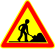# IllogiScript

 This is a decent article, but it's not enough for a programming language.
 This article is burly men unfolding umbrellas.  Maybe you should help it on its way.IllogiScript is a programming language especially made for Illogicopedia.

## Basics

```DEFINE X
START
X = Lel.
#Sets X to lel.
PRINT X
END
```

`DEFINE` is used to define variables.

`START` and `END` are pretty self-explanatory, they define the main function.

We define `X` and set it from `NULL` to the "Lel.".

`PRINT` is the way you put data on the final page, like straight out of python.

Therefore, this function will return "Lel.".

Also, `#`s are used for comments.

## Hax

```DEFINE X
START
X = GETWORKINGWINCODE(IllogiGames:The Maze)
PRINT X
END
```

The `GETWORKINGWINCODE` function is the easiest way to hack. It gets you the fol-proof way to win the game, even if the final win page is protected somehow.

Therefore, this code will print the win code of IllogiGames:The Maze.

You can also use the `WIN` function to quickly get the win page of a game, it'll be faster but it may not always work.

```START
PRINT WIN(IllogiGames:The Rain of Dead Cats)
END
```

Like here.

## Functions

```FUNC F = PARAMS(X) CODE(
PRINT X
)
START
F(10)
END
```

Custom functions are created with the `FUNC` keyword.

The `PARAMS` keyword will define the parameters of the funtion, and the `CODE` parameter defines the function itself.

## Math

```START
PRINT MATH(2+2)
END
```

Math also works, but it's in a function.

Because math follows some of the most basic math rules, this would return 5.

## Data types

There is no data types except for `NULL` numbers and strings. When a variable is defined, it's `NULL`. When a value is given, it beomes a string, and when the string matches the conditions of a number (ONLY 0-9 allowed), it becomes available for maths.

```DEFINE X
START
PRINT X
#prints NULL
X = String
PRINT X
#prints String
X = 123
PRINT MATH(X+1)
#prints 138
X = 123,456
PRINT MATH(X+1)
#prints ERROR: Can't do math with strings
```

There is a workaround to allow formatted numbers.

```DEFINE X
START
X = 123,456
FORMATRAW(X)
PRINT MATH(X+2)
#Would return 123471
```

Using the `FORMAT` (to format numbers) and `FORMATRAW` (to unformat numbers).